## 如何用Python创建一个简单的神经网络

• 输入层：接受传递数据
• 隐藏层
• 输出层
• 各层之间的权重
• 每个隐藏层都会有一个精心设计的激活函数，对于此教程，我们将会使用Sigmoid激活函数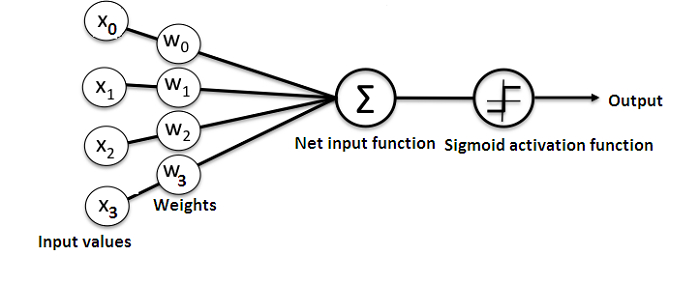### 问题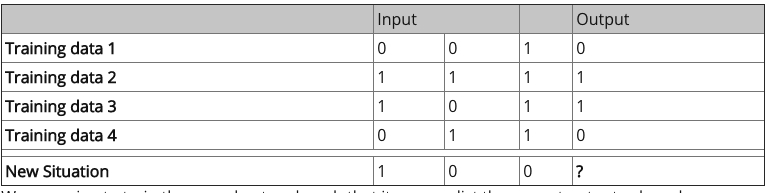### 创建一个神经网络类

• exp：用于生成自然指数
• array：用于生成矩阵
• dot：用于乘法矩阵
• random：用于生成随机数(注意：我们将对随机数进行播种以确保其有效分布)

#### 应用 Sigmoid 激活函数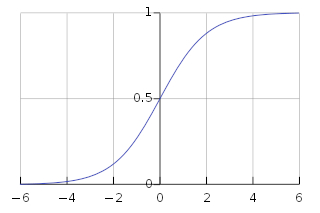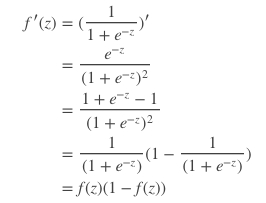#### 训练模型

• 从训练集获取输入数据，根据他们的权重进行调整，然后通过计算人工神经网络输出的方法将它们抽取出来
• 我们计算了反向传播的错误率，在这种情况下，它是神经元预测值和实际期望值之间的差异
• 根据误差程度，我们利用Error Weighted Derivative formula对权重进行微调
• 我们对这个过程重复15,000次，在每次迭代中都会同时处理整个训练集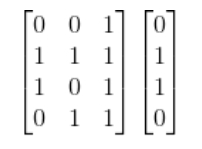### 总结

  1 2 3 4 5 6 7 8 9 10 11 12 13 14 15 16 17 18 19 20 21 22 23 24 25 26 27 28 29 30 31 32 33 34 35 36 37 38 39 40 41 42 43 44 45 46 47 48 49 50 51 52 53 54 55 56 57 58 59 60 61 62 63 64 65 66 67 68 69 70 71 72 73  import numpy as np class NeuralNetwork(): def __init__(self): # seeding for random number generation np.random.seed(1) # converting weights to a 3 by 1 matrix with values from -1 to 1 and mean of 0 self.synaptic_weights = 2 * np.random.random((3, 1)) - 1 def sigmoid(self, x): #applying the sigmoid function return 1 / (1 + np.exp(-x)) def sigmoid_derivative(self, x): #computing derivative to the Sigmoid function return x * (1 - x) def train(self, training_inputs, training_outputs, training_iterations): #training the model to make accurate predictions while adjusting weights continually for iteration in range(training_iterations): #siphon the training data via the neuron output = self.think(training_inputs) #computing error rate for back-propagation error = training_outputs - output #performing weight adjustments adjustments = np.dot(training_inputs.T, error * self.sigmoid_derivative(output)) self.synaptic_weights += adjustments def think(self, inputs): #passing the inputs via the neuron to get output #converting values to floats inputs = inputs.astype(float) output = self.sigmoid(np.dot(inputs, self.synaptic_weights)) return output if __name__ == "__main__": #initializing the neuron class neural_network = NeuralNetwork() print("Beginning Randomly Generated Weights: ") print(neural_network.synaptic_weights) #training data consisting of 4 examples--3 input values and 1 output training_inputs = np.array([[0,0,1], [1,1,1], [1,0,1], [0,1,1]]) training_outputs = np.array([[0,1,1,0]]).T #training taking place neural_network.train(training_inputs, training_outputs, 15000) print("Ending Weights After Training: ") print(neural_network.synaptic_weights) user_input_one = str(input("User Input One: ")) user_input_two = str(input("User Input Two: ")) user_input_three = str(input("User Input Three: ")) print("Considering New Situation: ", user_input_one, user_input_two, user_input_three) print("New Output data: ") print(neural_network.think(np.array([user_input_one, user_input_two, user_input_three]))) print("Wow, we did it!")